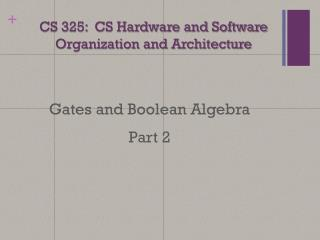Download PresentationCS 325: CS Hardware and Software Organization and Architecture# CS 325: CS Hardware and Software Organization and Architecture - PowerPoint PPT Presentation

Download Presentation##### CS 325: CS Hardware and Software Organization and Architecture

Download Policy: Content on the Website is provided to you AS IS for your information and personal use and may not be sold / licensed / shared on other websites without getting consent from its author. While downloading, if for some reason you are not able to download a presentation, the publisher may have deleted the file from their server.

- - - - - - - - - - - - - - - - - - - - - - - - - - - E N D - - - - - - - - - - - - - - - - - - - - - - - - - - -
##### Presentation Transcript

1. CS 325: CS Hardware and SoftwareOrganization and Architecture Gates and Boolean Algebra Part 2

2. Outline • Sum of Products (SOP) • Fan-in, Fan-out • Cascading to Reduce Inputs • Boolean Algebra Laws • Gate Reduction using Boolean Algebra

3. Circuits from SOP Functions • Why simplify circuits? • NAND and NOR gates are simpler (faster, smaller) than NOT AND and NOT OR. • Reduction in complexity when using a small number of gate types. • Goal: To implement circuit using a small complete set of operators. • NAND and NOR are both complete since any Boolean function can be implemented with either. • Faster to use small number of inputs to a gate (fan-in), and small number of gate inputs from a gate output (fan-out) • Typically, fan-in and fan-out < 10.

4. Logic Gate Fan-n and Fan-out

5. Gate Cascading to Reduce Inputs Implementing 3-input AND and OR functions with 2-input gates ABC = (AB)C A+B+C = (A+B)+C Implementing a 3-input NAND function with 2-input gates. NO! Correct

6. Basic Laws of Boolean Algebra • Boolean Algebra follows many algebra rules which can be used to make simpler circuits.

7. Basic Laws of Boolean Algebra • Boolean Algebra follows many algebra rules which can be used to make simpler circuits.

8. Basic Laws of Boolean Algebra • Boolean Algebra follows many algebra rules which can be used to make simpler circuits.

9. Basic Laws of Boolean Algebra • Boolean Algebra follows many algebra rules which can be used to make simpler circuits.

10. Basic Laws of Boolean Algebra • Boolean Algebra follows many algebra rules which can be used to make simpler circuits.

11. Basic Laws of Boolean Algebra • Boolean Algebra follows many algebra rules which can be used to make simpler circuits.

12. Basic Laws of Boolean Algebra • Boolean Algebra follows many algebra rules which can be used to make simpler circuits.

13. Basic Laws of Boolean Algebra • Boolean Algebra follows many algebra rules which can be used to make simpler circuits.

14. Basic Laws of Boolean Algebra • Boolean Algebra follows many algebra rules which can be used to make simpler circuits.

15. Basic Laws of Boolean Algebra • Boolean Algebra follows many algebra rules which can be used to make simpler circuits. • Example: AB + AC Three gates • = A(B + C), Distributive Law Two gates

16. Gate Reduction • AB + AC Three gates • = A(B + C), Distributive Law Two gates

17. Equivalent Gates/Symbols • Using Boolean Laws (identities), alternative symbols for some gates can be derived:

18. Functionally Complete Sets of Gates • Not all gate types are typically implemented in circuit design. • Simpler if only 1 or 2 types of gates are used. • A functionally complete set of gates means that any Boolean function can be implemented using only the gates in that set. • Examples of functionally complete sets: • AND, OR, NOT • AND, NOT • OR, NOT • NAND • NOR

19. NAND and NOR Completeness

20. Implement XOR with NANDs • Exclusive-OR (XOR) example: • Step 1: build truth table • Step 2: find SOP and build circuit using AND and OR.

21. Implement XOR with NANDs • Apply Boolean Algebra rules: so, De Morgan’s Law: so, • The last formula is 3 NAND gates.

22. Implement XOR with NANDs • Logic circuits implementing XOR:

23. Simplification • Boolean functions, and therefore circuits, can usually be manipulated using Boolean laws into simpler functions. Distributive Law Inverse Law Distributive Law Inverse Law Idempotent Law Identity Law • How to check for correctness?

24. Checking Logic for Correctness • We can check our solution using a truth table • Checking

25. Checking Logic for Correctness • We can check our solution using a truth table • Checking Correct

26. Another Example • Draw the logic gate diagram for the following Boolean function: • Circuit Reduction: Distributive Law Idempotent Law Idempotent Law Distributive Law • Check with Truth table for correctness.

27. Checking Logic for Correctness • We can check our solution using a truth table • Checking

28. Checking Logic for Correctness • We can check our solution using a truth table • Checking Correct

29. Another Example • Draw the logic gate diagram for the following Boolean function: • Circuit Reduction: Distributive Law Idempotent Law Absorption Law Distributive Law Idempotent Law Absorption Law Absorption Law • Check with Truth table for correctness.